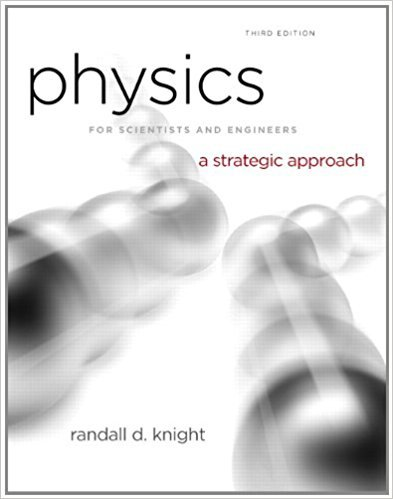×
Get Full Access to Physics For Scientists And Engineers: A Strategic Approach With Modern Physics - 3 Edition - Chapter 6 - Problem 36p
Get Full Access to Physics For Scientists And Engineers: A Strategic Approach With Modern Physics - 3 Edition - Chapter 6 - Problem 36p

×

# The piston of a machine exerts a constant force on a ballISBN: 9780321740908 69

## Solution for problem 36P Chapter 6

Physics for Scientists and Engineers: A Strategic Approach with Modern Physics | 3rd Edition

• Textbook Solutions
• 2901 Step-by-step solutions solved by professors and subject experts
• Get 24/7 help from StudySoup virtual teaching assistantsPhysics for Scientists and Engineers: A Strategic Approach with Modern Physics | 3rd Edition

4 5 1 392 Reviews
27
5
Problem 36P

Problem 36P

The piston of a machine exerts a constant force on a ball as it moves horizontally through a distance of 15 cm. You use a motion detector to measure the speed of five different balls as they come off the piston; the data are shown below. Use theory to find two quantities that, when graphed, should give a straight line. Then use the graph to find the size of the piston’s force.

 Mass (g) Speed (m/s) 200 9.4 400 6.3 600 5.2 800 4.9 1000 4.0

Step-by-Step Solution:

Solution 36P

Step 1 of 4

We are required to plot a graph from the values given and find the magnitude of the piston’s force.

Step 2 of 4

Step 3 of 4

##### ISBN: 9780321740908

This full solution covers the following key subjects: use, piston, speed, Force, Find. This expansive textbook survival guide covers 17 chapters, and 1439 solutions. This textbook survival guide was created for the textbook: Physics for Scientists and Engineers: A Strategic Approach with Modern Physics, edition: 3. The full step-by-step solution to problem: 36P from chapter: 6 was answered by , our top Physics solution expert on 08/30/17, 04:34AM. Since the solution to 36P from 6 chapter was answered, more than 4225 students have viewed the full step-by-step answer. The answer to “The piston of a machine exerts a constant force on a ball as it moves horizontally through a distance of 15 cm. You use a motion detector to measure the speed of five different balls as they come off the piston; the data are shown below. Use theory to find two quantities that, when graphed, should give a straight line. Then use the graph to find the size of the piston’s force.Mass (g)Speed (m/s)2009.44006.36005.28004.910004.0” is broken down into a number of easy to follow steps, and 74 words. Physics for Scientists and Engineers: A Strategic Approach with Modern Physics was written by and is associated to the ISBN: 9780321740908.

Unlock Textbook Solution